# Function TransformationsJust like Transformations in Geometry, we can move and resize the graphs of functions

Let us start with a function, in this case it is f(x) = x2, but it could be anything:f(x) = x2

Here are some simple things we can do to move or scale it on the graph:

### We can move it up or down by adding a constant to the y-value: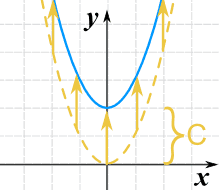g(x) = x2 + C

Note: to move the line down, we use a negative value for C.

• C > 0 moves it up
• C < 0 moves it down

### We can move it left or right by adding a constant to the x-value: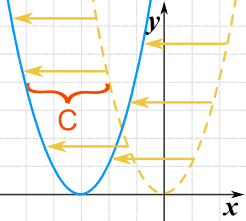g(x) = (x+C)2

Adding C moves the function to the left (the negative direction).

Why? Well imagine you will inherit a fortune when your age=25. If you change that to (age+4) = 25 then you will get it when you are 21. Adding 4 made it happen earlier.

• C > 0 moves it left
• C < 0 moves it right

BUT we must add C wherever x appears in the function (we are substituting x+C for x).

### Example: the function v(x) = x3 - x2 + 4x

To move C spaces to the left, add C to x wherever x appears:

w(x) = (x + C)3 − (x + C)2 + 4(x + C)

An easy way to remember what happens to the graph when we add a constant:

add to y to go high
add to x to go left

### We can stretch or compress it in the y-direction by multiplying the whole function by a constant.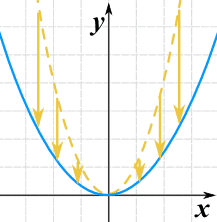g(x) = 0.35(x2)

• C > 1 stretches it
• 0 < C < 1 compresses it

### We can stretch or compress it in the x-direction by multiplying x by a constant.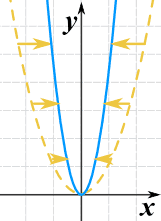g(x) = (2x)2

• C > 1 compresses it
• 0 < C < 1 stretches it

Note that (unlike for the y-direction), bigger values cause more compression.

### We can flip it upside down by multiplying the whole function by −1:g(x) = −(x2)

This is also called reflection about the x-axis (the axis where y=0)

We can combine a negative value with a scaling:

Example: multiplying by −2 will flip it upside down AND stretch it in the y-direction.

### We can flip it left-right by multiplying the x-value by −1:g(x) = (−x)2

It really does flip it left and right! But you can't see it, because x2 is symmetrical about the y-axis. So here is another example using √(x):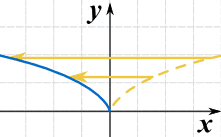g(x) = √(−x)

This is also called reflection about the y-axis (the axis where x=0)

## Summary

 y = f(x) + C C > 0 moves it up C < 0 moves it down y = f(x + C) C > 0 moves it left C < 0 moves it right y = Cf(x) C > 1 stretches it in the y-direction 0 < C < 1 compresses it y = f(Cx) C > 1 compresses it in the x-direction 0 < C < 1 stretches it y = −f(x) Reflects it about x-axis y = f(−x) Reflects it about y-axis

## Examples

### Example: the function g(x) = 1/x

Here are some things we can do:

Move 2 spaces up:h(x) = 1/x + 2
Move 3 spaces down:h(x) = 1/x − 3
Move 4 spaces right:h(x) = 1/(x−4) graph
Move 5 spaces left:h(x) = 1/(x+5)
Stretch it by 2 in the y-direction:h(x) = 2/x
Compress it by 3 in the x-direction:h(x) = 1/(3x)
Flip it upside down:h(x) = −1/x

### Example: the function v(x) = x3 − 4x

Here are some things we can do:

Move 2 spaces up:w(x) = x3 − 4x + 2
Move 3 spaces down:w(x) = x3 − 4x − 3
Move 4 spaces right:w(x) = (x−4)3 − 4(x−4)
Move 5 spaces left:w(x) = (x+5)3 − 4(x+5)  graph
Stretch it by 2 in the y-direction:w(x) = 2(x3 − 4x)
= 2x3 − 8x
Compress it by 3 in the x-direction:w(x) = (3x)3 − 4(3x)
= 27x3 − 12x
Flip it upside down:w(x) = −x3 + 4x

## All In One ... !

We can do all transformation in one go using this: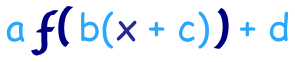a is vertical stretch/compression

• |a| > 1 stretches
• |a| < 1 compresses
• a < 0 flips the graph upside down

b is horizontal stretch/compression

• |b| > 1 compresses
• |b| < 1 stretches
• b < 0 flips the graph left-right

c is horizontal shift

• c < 0 shifts to the right
• c > 0 shifts to the left

d is vertical shift

• d > 0 shifts upward
• d < 0 shifts downward

### Example: 2√(x+1)+1

a=2, c=1, d=1

So it takes the square root function, and then

• Stretches it by 2 in the y-direction
• Shifts it left 1, and
• Shifts it up 1

Play with this graph